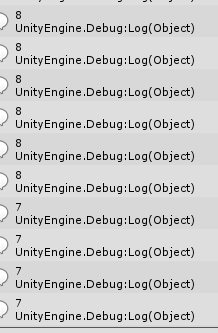# Im doing a Countdown on Debug. and it works but the numbers repeat.

I would like that only appears 1 time..

``````using UnityEngine;
using System.Collections;

public class CuentaAtras : MonoBehaviour {

float currentTime = 0f;
float StartingTime = 10f;

void Start () {
currentTime = StartingTime;

}
void Update()
{

currentTime -= Time.deltaTime;
Debug.Log(currentTime.ToString("f0"));
if (currentTime <= 0)
{
currentTime = 0;
Debug.Log(currentTime);
Debug.Log("Se acabo el tiempo");
enabled = false;
}
}
}
``````

It is repeating because it is a float, but you have formatted it to show just the whole number without the decimals. The actual value is hidden, if you didn’t format it with ToString(“F0”); you would be getting decimals like 8.954, 8.606, etc… If you want to do a countdown from 10 to 0 and you want to use a float to do so. Just check if the value is the same as the last frame and if it is, don’t use it:

`````` using UnityEngine;
using System.Collections;

public class CuentaAtras : MonoBehaviour {

float currentTime = 0f;
float StartingTime = 10f;
string timeLastFrame = “0”;

void Start () {
currentTime = StartingTime;

}
void Update()
{

currentTime -= Time.deltaTime;
if(timeLastFrame != currentTime.ToString(“f0”)){

timeLastFrame = currentTime.ToString(“f0”);
Debug.Log(timeLastFrame);
}
if (currentTime <= 0)
{
currentTime = 0;
Debug.Log(currentTime);
Debug.Log("Se acabo el tiempo");
enabled = false;
}
}
}
``````